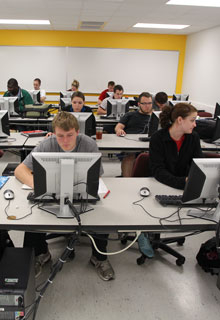# Applied Mathematics Track

## Connecting to other disciplines through mathematical modeling

Applied mathematics is a branch of mathematics that focuses on the application of mathematical theory and methods to other areas such as physics, biology, medicine, computer sciences and life sciences.

A central part of applied mathematics is that of mathematical modeling, the process through which a real-world problem is transformed into a mathematical problem. In Missouri State’s applied mathematics track, students receive not only a comprehensive mathematics education, but also a wide range of classical and current real-world applications for math.

Within this track, math majors must take MTH 421 (Numerical Analysis I) and MTH 580 (Applied Mathematics). They must also select an additional six hours from the following courses:

• MTH 422 Numerical Analysis II
• MTH 507 Introduction to Partial Differential Equations
• MTH 537 Applied Abstract Algebra
• MTH 543 Stochastic Modeling
• MTH 570 Combinatorial Analysis